# PSAT Math : How to find the length of the hypotenuse of a right triangle : Pythagorean Theorem

## Example Questions

← Previous 1 3 4 5 6

### Example Question #461 : Geometry

What is the hypotenuse of a right triangle with sides 5 and 8?

√89

8√13

5√4

12

15

√89

Explanation:

Because this is a right triangle, we can use the Pythagorean Theorem which says a2 + b2 = c2, or the squares of the two sides of a right triangle must equal the square of the hypotenuse. Here we have a = 5 and b = 8.

a2 + b2 = c2

52 + 82 = c2

25 + 64 = c2

89 = c2

c = √89

### Example Question #1 : How To Find The Length Of The Hypotenuse Of A Right Triangle : Pythagorean Theorem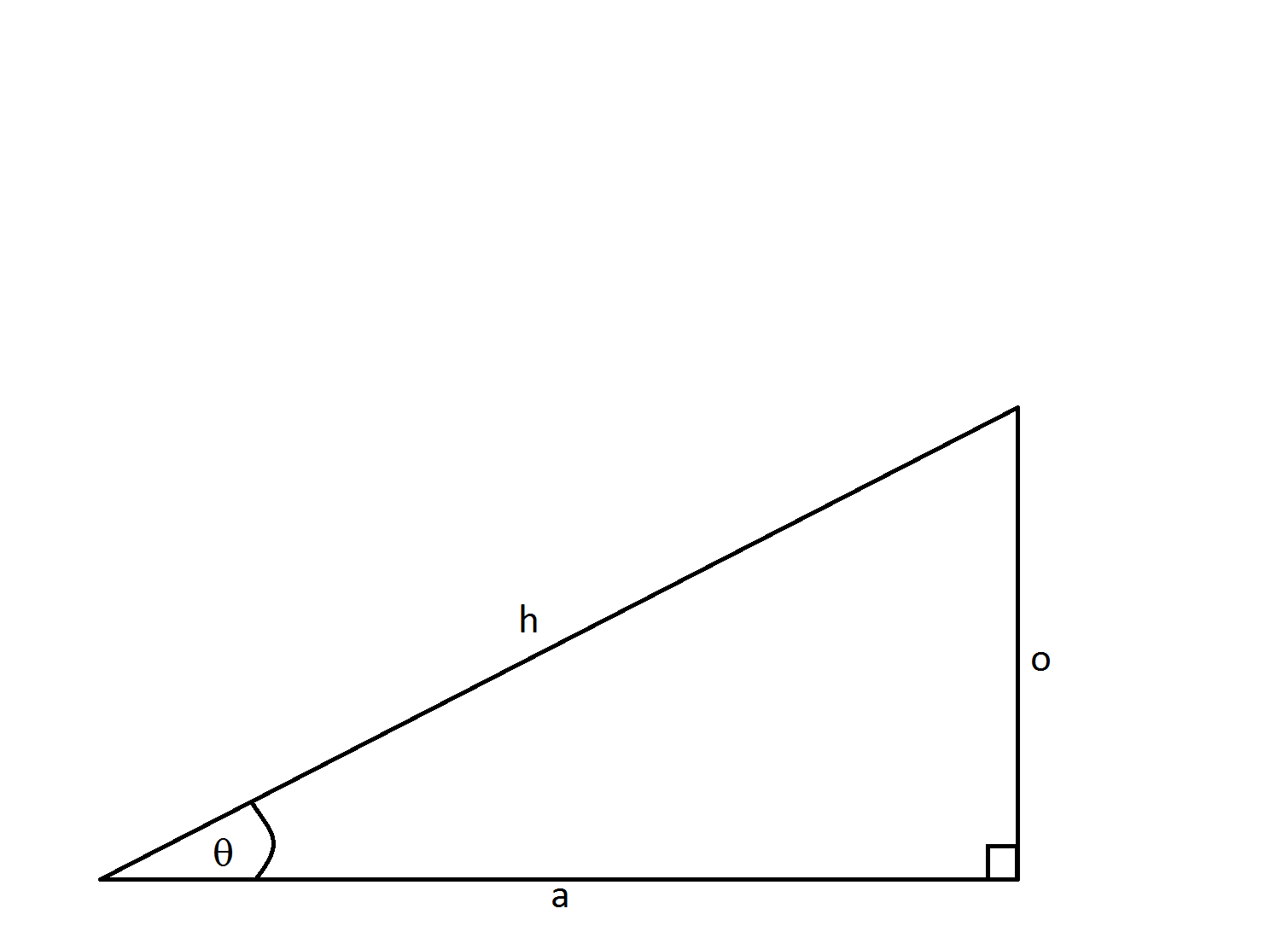If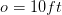and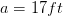, how long is side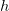?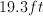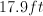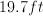Not enough information to solveExplanation:

This problem is solved using the Pythagorean theorem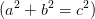.  In this formulaandare the legs of the right triangle whileis the hypotenuse.

Using the labels of our triangle we have: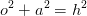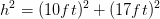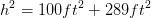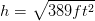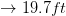### Example Question #951 : High School Math

If one of the short sides of a 45-45-90 triangle equals 5, how long is the hypotenuse?

√15

5√2

5

√10

π

5√2

Explanation:

Using the Pythagorean theorem, x2 + y2 = h2. And since it is a 45-45-90 triangle the two short sides are equal. Therefore 52 + 52 = h2 .  Multiplied out 25 + 25 = h2.

Therefore h2 = 50, so h = √50 = √2 * √25 or 5√2.

### Example Question #1 : How To Find The Length Of The Hypotenuse Of A Right Triangle : Pythagorean Theorem

The height of a right circular cylinder is 10 inches and the diameter of its base is 6 inches. What is the distance from a point on the edge of the base to the center of the entire cylinder?

√(43)/2

3π/4

None of the other answers

√(34)

4π/5

√(34)

Explanation:

The best thing to do here is to draw diagram and draw the appropiate triangle for what is being asked. It does not matter where you place your point on the base because any point will produce the same result.  We know that the center of the base of the cylinder is 3 inches away from the base (6/2). We also know that the center of the cylinder is 5 inches from the base of the cylinder (10/2). So we have a right triangle with a height of 5 inches and a base of 3 inches. So using the Pythagorean Theorem 3+ 5= c2. 34 = c2, c = √(34).

### Example Question #1 : How To Find The Length Of The Hypotenuse Of A Right Triangle : Pythagorean Theorem

A right triangle with sides A, B, C and respective angles a, b, c has the following measurements.

Side A = 3in. Side B = 4in. What is the length of side C?

25

5

9

6

7

5

Explanation:

The correct answer is 5. The pythagorean theorem states that a+ b= c2. So in this case 3+ 4= C2. So C= 25 and C = 5. This is also an example of the common 3-4-5 triangle.

### Example Question #1 : How To Find The Length Of The Hypotenuse Of A Right Triangle : Pythagorean Theorem

The lengths of the three sides of a right triangle form a set of consecutive even integers when arranged from least to greatest. If the second largest side has a length of x, then which of the following equations could be used to solve for x?

(x – 1)2 + x2 = (x + 1)2

(x – 2) + x = (x + 2)

(x – 2)2 + x2 = (x + 2)2

x 2 + (x + 2)2 = (x + 4)2

(x + 2)2 + (x – 2)2 = x2

(x – 2)2 + x2 = (x + 2)2

Explanation:

We are told that the lengths form a series of consecutive even integers. Because even integers are two units apart, the side lengths must differ by two. In other words, the largest side length is two greater than the second largest, and the second largest length is two greater than the smallest length.

The second largest length is equal to x. The second largest length must thus be two less than the largest length. We could represent the largest length as x + 2.

Similarly, the second largest length is two larger than the smallest length, which we could thus represent as x – 2.

To summarize, the lengths of the triangle (in terms of x) are x – 2, x, and x + 2.

In order to solve for x, we can make use of the fact that the triangle is a right triangle. If we apply the Pythagorean Theorem, we can set up an equation that could be used to solve for x. The Pythagorean Theorem states that if a and b are the lengths of the legs of the triangle, and c is the length of the hypotenuse, then the following is true:

a2 + b2 = c2

In this particular case, the two legs of our triangle are x – 2 and x, since the legs are the two smallest sides; therefore, we can say that a = x – 2, and b = x. Lastly, we can say c = x + 2, since x + 2 is the length of the hypotenuse. Subsituting these values for a, b, and c into the Pythagorean Theorem yields the following:

(x – 2)2 + x2 = (x + 2)2

The answer is (x – 2)2 + x2 = (x + 2)2.

### Example Question #1 : How To Find The Length Of The Hypotenuse Of A Right Triangle : Pythagorean Theorem

What is the hypotenuse of a right triangle with sides 5 and 8?

15

√89

5√4

8√13

12

√89

Explanation:

Because this is a right triangle, we can use the Pythagorean Theorem which says a2 + b2 = c2, or the squares of the two sides of a right triangle must equal the square of the hypotenuse. Here we have a = 5 and b = 8.

a2 + b2 = c2

52 + 82 = c2

25 + 64 = c2

89 = c2

c = √89

### Example Question #1 : How To Find The Length Of The Hypotenuse Of A Right Triangle : Pythagorean Theorem

A right triangle has side lengths of 21 and 72. What is the length of the hypotenuse?

67

75

70

80

84

75

Explanation:

By the Pythagorean Theorem, 212 + 722 = hyp2. Then hyp2 = 5625, and the hypotenuse = 75. If you didn't know how to figure out that 752 = 5625, that's okay. Look at the answer choices. We could easily have squared them and chosen the answer choice that, when squared, equals 5625.

### Example Question #1 : How To Find The Length Of The Hypotenuse Of A Right Triangle : Pythagorean Theorem

Sam and John both start at the same point.  Sam walks 30 feet north while John walks 40 feet west.  How far apart are they at their new locations?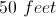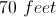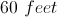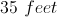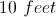Explanation:

Sam and John have walked at right angles to each other, so the distance between them is the hypotenuse of a triangle.  The distance can be found using the Pythagorean Theorem.

### Example Question #1 : How To Find The Length Of The Hypotenuse Of A Right Triangle : Pythagorean Theorem

Which of these triangles cannot have a right angle?

5, 12, 13

6,7,12

2, 2, 2√2

√2, √2, 2

9, 12, 15